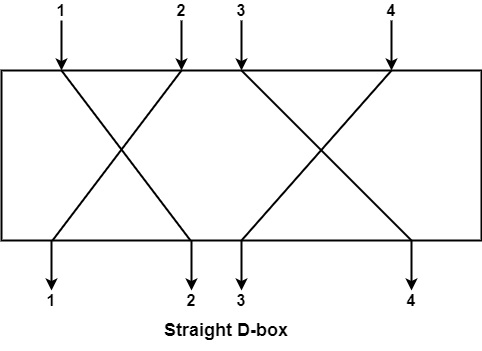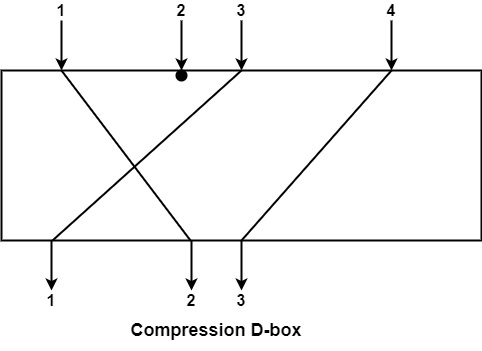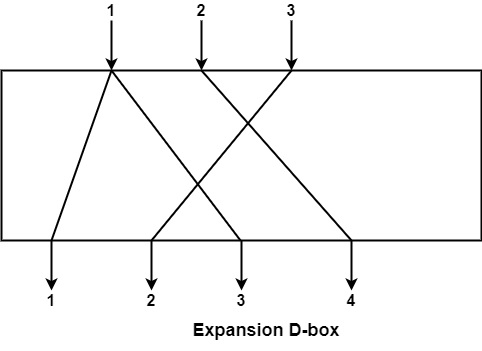# What are the components of Modern Block Cipher in Information Security?

A modern block cipher is a cipher which encrypts m-bit block of plaintext and decrypts m-bit block of ciphertext. For encryption or decryption, modern block cipher facilitate a K bit key and the decryption algorithm should be inverse of encryption algorithms and for both encryption and decryption similar key is used.

A block cipher works on a plaintext block of n bits to make a cipher text block of n bits. There are possible multiple plaintext blocks and, for the encryption to be reversible (i.e., for decryption to be applicable), each should create a unique cipher text block. Such transformation is known as reversible, or non-singular.

Block cipher modes of operation have been produced to delete the chance of encrypting identical blocks of text the similar method, the ciphertext formed from the previous encrypted block is used to the next block. A block of bits is known as an initialization vector (IV).

There are various components of Modern Block Cipher which are as follows −

• D-boxes − A D-box is a permutation box having similar features as traditional transposition ciphers. D-boxes transpose bits. There are three types of D-boxes which are as follows −

Straight D-box − It creates n inputs, permutes them and supports n outputs. In this, the second input after permutation is the first to be outputted. The first letter in input is permuted to second place, third on fourth place and fourth on third place. There are n! Possible way of mapping in D-box.Compression D-box − This is a D-box with n inputs and m outputs, where m<n. There are various inputs are blocked and do not reach the output. Compression D-boxes are used when it is required to permute bits and at the similar time reduce the number of bits for the next stage.Expansion D-box − This is a D-box with n inputs and m outputs, where m >n i.e., there are various inputs are connected to more than one output it is used when it is required to transpose bits and the same increase the multiple bits for the next stage.• S-boxes − These are substitution boxes same to the substitution cipher. The input to an S-box can be a n-bit word but the output can be a m-bit word, where m and n are not essentially the same.

• Circular Shift − It can also discovered in modern block ciphers, it can be such as leftshift or right-shift. In the circular left shift, shift each bit in n-bit word with m position to the left and the leftmost m-bits are deleted from the left and become the rightmost bits.